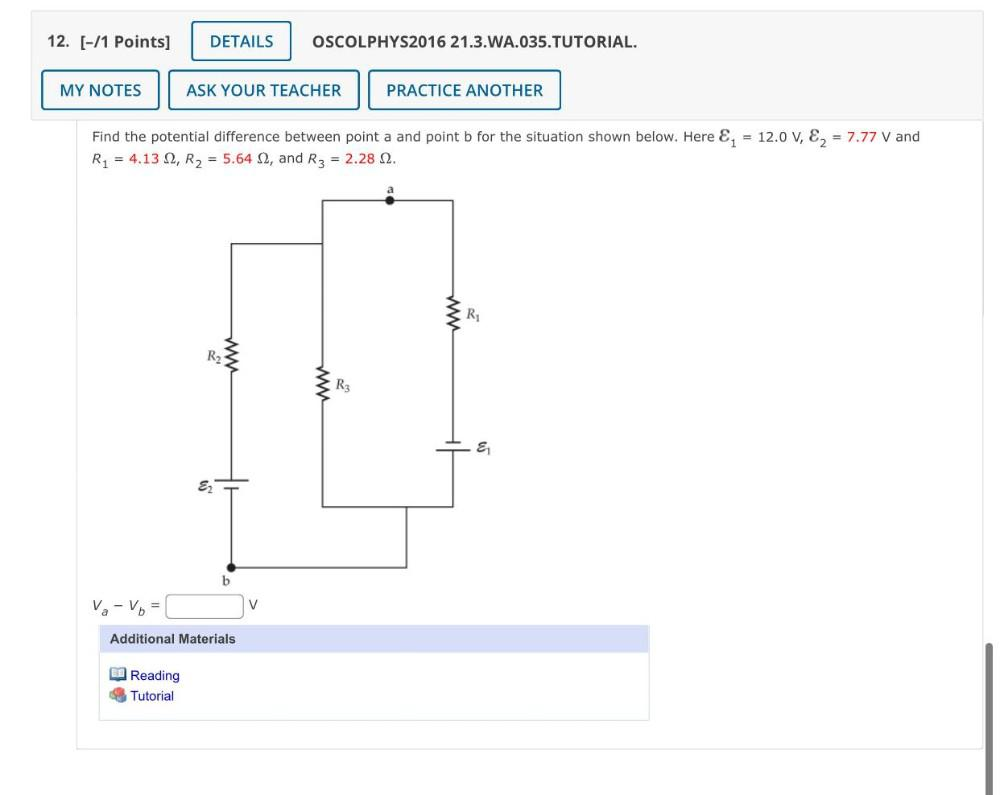Question:

# 12. [-/1 Points] DETAILS OSCOLPHYS2016 21.3.WA.035.TUTORIAL. MY NOTES ASK YOUR TEACHER PRACTICE ANOTHER Find the potential diffe12. [-/1 Points] DETAILS OSCOLPHYS2016 21.3.WA.035.TUTORIAL. MY NOTES ASK YOUR TEACHER PRACTICE ANOTHER Find the potential difference between point a and point b for the situation shown below. Here &; = 12.0 V, E2 = 7.77 V and R1 = 4.132, R2 = 5.642, and R3 = 2.28 2. R1 R2 www R3 E b va - Vo = V Additional Materials Reading Tutorial